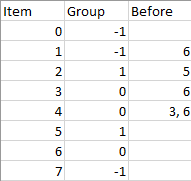| English | 简体中文 |

# 1203. Sort Items by Groups Respecting Dependencies

## Description

There are n items each belonging to zero or one of m groups where group[i] is the group that the i-th item belongs to and it's equal to -1 if the i-th item belongs to no group. The items and the groups are zero indexed. A group can have no item belonging to it.

Return a sorted list of the items such that:

• The items that belong to the same group are next to each other in the sorted list.
• There are some relations between these items where beforeItems[i] is a list containing all the items that should come before the i-th item in the sorted array (to the left of the i-th item).

Return any solution if there is more than one solution and return an empty list if there is no solution.

Example 1:Input: n = 8, m = 2, group = [-1,-1,1,0,0,1,0,-1], beforeItems = [[],,,,[3,6],[],[],[]]
Output: [6,3,4,1,5,2,0,7]


Example 2:

Input: n = 8, m = 2, group = [-1,-1,1,0,0,1,0,-1], beforeItems = [[],,,,,[],,[]]
Output: []
Explanation: This is the same as example 1 except that 4 needs to be before 6 in the sorted list.


Constraints:

• 1 <= m <= n <= 3 * 104
• group.length == beforeItems.length == n
• -1 <= group[i] <= m - 1
• 0 <= beforeItems[i].length <= n - 1
• 0 <= beforeItems[i][j] <= n - 1
• i != beforeItems[i][j]
• beforeItems[i] does not contain duplicates elements.